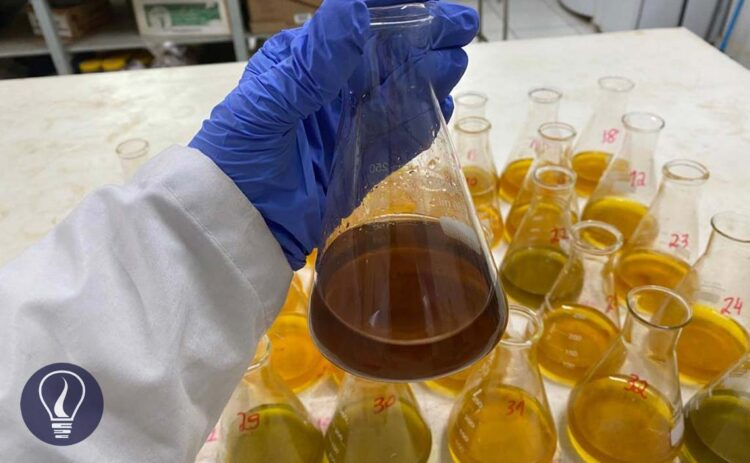More results...

Generic selectors
Exact matches only
Search in title
Search in content
Post Type Selectors

# Complexometric Titrations (Chelatometry) - Principles and ExamplesB.Sc (Hons, USJ) (Polymer Science and Technology, Chemistry, Physics)
Categories
Tags :
Last Updated On : March 10, 2023
Published Date : March 10, 2023

Titration methods are commonly used in laboratories to determine an unknown concentration of a substance. There are four types of titrations. They are,

• Acid-base titrations,
• Redox titrations,
• Precipitation titrations
• Complexometric titrations.

## What is complexometric titration?

Many cations form complexes with different substances. A cation can be known as a Lewis acid. That means a cation is accepting electron pair. In an aqueous medium, cations are donated electrons by different substances and form a coordinate bond also known as a dative covalent bond.

In a coordinate bond, both two electrons are donated by one molecule or an ion. These molecules or ions are called ligands. The number of ligands that have bonded with the cation depends on the coordination number of the cation.

The above principle is used in Chelatometry to identify unknown concentrations of complex-forming ions.

In a complexometric titration, a colored ion complex is formed, and it is used to determine the endpoint.

## Formation constant (Kf) of an ion complex

The formation constant also known as the stability constant is an expression of the formation of a metal ion complex. We can describe it using an example as follows.

In an aqueous medium containing Ammonia (NH3) and Cu2+ ions, an ion complex is formed with four NH3 molecules and one Cu2+ ion. Ammonia acts as a Lewis base and it shares one pair of electrons with the Cu2+ ion. Cu2+ ion acts as a Lewis base here. The complex has tetrahedral symmetry, and it is deep blue in color.

We can write the formation constant for the above equilibrium.

Sometimes the ion complex is formed as a stepwise process.

As an example, Ammonia will be complex with silver ion (Ag+) in a stepwise fashion. In such formation, the formation constant can be obtained by the product formation constants of each step.

• The first step of formation,

• The second step of formation,

• Overall formation of the complex,

If the formation constant is high, that means the ion complex has high stability.

We can write the formation expression in the opposite direction as the complex dissociates. Then all the concentration terms are inverted. As we write the equilibrium constant for the dissociation of the complex, the equilibrium constant would be the instability constant (Ki) also known as the dissociation constant (Kd)

## How to solve questions that involve complexometric titrations?

Question 01

In aqueous medium, Silver ion forms a stable 1:1 complex with triethylenetetramine, called “trien” [NH2(CH2)2NH(CH2)2NH(CH2)2NH2]. Calculate the silver ion concentration at equilibrium when 25 mL of 0.010 M silver nitrate is added to 50 mL of 0.015 M trien. The stability constant of the complex is 5.0 × 107 mol-1 dm3.

Solution

The formation constant of the above complex is so high. Therefore, the equilibrium tends to the formation of the complex. We can assume that all Ag+ ions will react with trien to form the complex. The complex is formed as follows.

If we take the total volume of the solution as “V”, we can calculate the initial concentrations (before the complex is formed) of each component.

Here, we have assumed that all the Ag+ ions are reacted with trien to form the complex.

Therefore, after the complex is formed there are no Ag+ ions remaining. But there is an excess amount of trien in the medium.

So, trien will remain in the medium. Ag+ ions and trien will react 1:1 stoichiometric ratio.

Therefore, the remaining trien concentration can be found by subtracting the Ag+ concentration from the initial trien concentration. We can calculate the concentrations of each component after the complex is formed as follows.

After the complex is formed, some amount of the complex will dissociate and become equilibrium. Let’s take the dissociated concentration of the complex as “x”. We can write the equilibrium concentrations of each component as follows.

Let’s summarize the above values as follows,

When compared to the trien concentration and the concentration of the complex at the equilibrium, x is negligible. So, we can simplify the above expression as follows.## References and Attributes

Daniel C. Harris, Quantitative Chemical Analysis; Ninth edition

Douglas A. Skoog, Donald M. West, F. James Holler, Stanley R. Crouch, Fundamentals of Analytical Chemistry; Ninth edition

### Figures:

The cover image was created using an image by Dra Taciara Zborowski Horst, licensed under CC0, via Wikimedia Commons